# Spring Model and Properties of Crystals

Looking at Some Everyday SpringLet's look at two atoms that are connected by a spring. One atom we assume to be somehow fixed; it is like an unmovable wall as shown below. The other atom has the mass m and the spring has the spring constant ks (just wait). We apply some force F in the direction of the bond / spring that causes some deflection or change of position x with respect to the zero point, the position assumed without a force.Never mind that the spring shown below is horizontal. Gravity, the force that would make a big mass on a real spring point downwards, can be completely neglected if we look at forces between atoms.For some technical reasons we choose the signs in such a way that a negative force will make the spring longer, i.e. it cause some positive deflection x. If you don't like the signs, change them and redraw the picture. They are completely meaningless anyway, we just have to adopt some system so we can talk unambiguously like scientists and not like politicians or lawyers. (Is the sign of the national debt positive or negative? In a mathematical sense or a good / bad sense?).An ideal High-School spring obeys a linear force F - deflection x law, called Hooke's law, or

x  =  – 1
ks
·  FThe spring constant ks = –F/x describes the actual spring and not the material the spring is made from. If we want to assign spring properties to the properties of the material the spring is made from, and not to the size of the spring material, it would be a good idea to look at some specific spring constant that is somehow independent of the size. That would be easy enough but I won't do it because we already did that. We replace forces by stress, elongation by strain - and the spring constant then is called Young's modulus. A large spring constant (or Youngs's modulus) means that you need large forces to elongate or squeeze your material somewhat; we have a stiff spring.There are two important things to realize at this point:
1. An ideal spring, described by Hooke's law above, can be squeezed or pulled out to any lenght you like.
2. There is no such thing as an ideal spring.If you squeeze a real spring to the point where all its coils touch each other, it's all over. You have to increase the force a lot to cause just a tiny little bit of additional deflection. We note: All springs get extremely "hard" if shortened enough.
If you go in the other direction and elongate your spring, it's all over as soon as you stretched the coil to a straight line. Sometimes (but not always) you destroyed the whole thing by then and you can pull it to larger lengths with little additional force. The spring then becomes "soft".All of this is shown below. In the force vs. deflection diagram (red line), an ideal spring is just a straight line through the origin. A real spring as described above deviates as shown for large deflections in both directions. Getting harder means the the curve gets steeper, getting softer means it flattens out.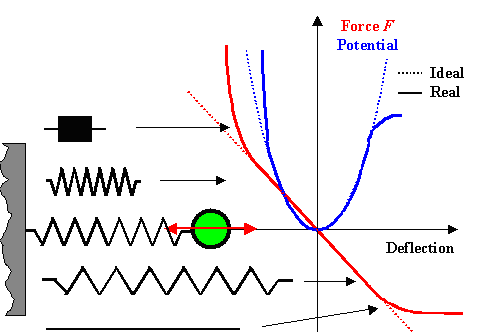Analyzing a real springForces are nice, energies are nicer. If we look at the work or energy necessary to stretch or compress the spring to a certain deflection, we simply need to remember the definition of work = force times distance = force times deflection x = potential (energy) U in this case. Since the forces in this case are not constant but change with the deflection,we have to sum up and get

U(x)  = x ó õ 0 x ó õ 0We have a perfect parabola for the ideal spring and a distorted parabola "with wings" for the real spring. We cannot write down an equation for the parabola with wings, but the cool thing about potential energy curves is that we often don't need to calculate them in detail. Quite often we can make very good guesses on what they must look like, as we just did for a real spring. There is no need to write down an equation because potential curves; if we have them, can tell us a lot just so. Let's see how this works by using some model potential that approximates a real spring in the picture below.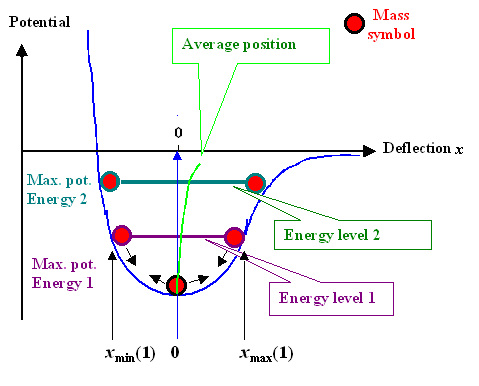Analyzing a real bond "spring"The figure above shows what one can see directly as soon as we have the graph of some potential well, as we will now call any potential energy curve (simply called potential) with some kind of minimum. All you need to do is to symbolize the mass by some sphere or whatever, and then draw its position for some deflection. What you see directly is:
1. If your mass is not at the potential minimum, it "wants" to move down into the minimum of the well, just as a real sphere in a real well or bowl, shaped like this potential, would do.
2. The force that tries to send the mass down into the minimum is proportional to the slope at the point where the mass is. The steeper, the more force, always in the "down" direction. That's what you feel when you stand on some slope on roller skates. On steep slopes you feel more force and you accelerate (proportional to the force, heed Newton's first law) faster.
3. The mass has only potential energy at the point where it is originally held. How much is directly given by the position. If you let go, it will move down, run through the potential minimum with maximum speed, and then move up on the other side to exactly the same potential energy it had originally (we assume that there is no friction). At the zero point the mass has only kinetic energy that must be exactly as large as the potential energy at its starting point. At intermediate points is has a mix of kinetic and potential energy but the sum total is always constant and equal to the potential energy at the starting point.
4. A horizontal line at the maximum potential energy thus symbolizes the amount of the total energy or the energy level that is characteristic for the the oscillation that results when you let the mass go. The energy level gives the total energy, which is concerved and thus the same at any position.
5. The average position of the mass is in the middle of the line symbolizing the energy level since the (oscillating) mass is just as often to the left as to the right. Since our potential gets flatter to the right, the average position shifts increasingly to the right of the center line at x = 0 for larger and larger deflections.Fascinating, isn't it? No? OK, then let's make the big step:

Bonds Between Atoms and the Common SpringThe picture above can be see as the potential of a trivial common spring, for example the one from the inside of your ball point writer. It can also be seen as the potential between two atoms in a crystal. As long as we don't care for numbers or details, the curves look exactly the same.We thus can treat the bonds between any two atoms in a crystal as if they would be a common real spring with the proper potential. Of course, this potential is quite different in numbers and details between iron and iron atoms, or iron and carbon atoms or A and B atoms - but qualitatively it always looks more or less as shown above.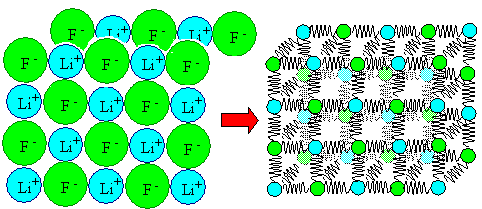Spring model of a simple (e.g. LiF) crystalEverything stated above thus is also valid for the atoms in a crystal. In particular, they vibrate around their average position with an energy that is typical for the crystal as a whole and thus (on average) also for each and every atom.The thing to realize here and now is that the word temperature for any crystal is just another word for ""average energy contained in the vibrations of the crystal atoms". The energy levels 1 and 2 in the potential figure above thus simply correspond to different temperatures T1 < T2 if we consider a crystal.If we have the potential of the bonding springs, we have a lot of crystal properties covered. Below is another picture of such a potential with a few more parameters that come in handy as soon as we get serious about properties.The distance between the atom is now called r. Imagining the atom as small spheres with radius r0 (numbers for most atoms via this link), the distance between two of them just touching each other then is about roughly 2r0 or around 0.3 nm. If the atoms are at rest, i.e. at extremely low temperature, they all are "sitting" right at the deepest point of the potential with an equilibrium distance of r0, a parameter that is extremely easy to measure and thus a known quantity.The depth of the potential well is the binding energy U0. If you want to rip the atoms apart, you have to increase the distance between them to a large value, and that means, as the potential picture directly shows, that you must invest the energy U0.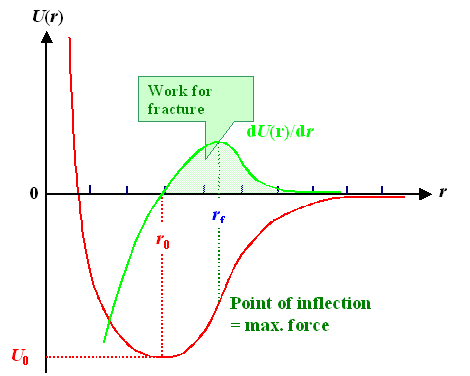Binding potential, some parameters, and the force between the atomsBefore I go on, just to show off, I'll give you some equations. The binding potential in a rather general but still simple way can be written as

U(r)  = –  A
r n
+   B
r m
A  =  U0 · r0n  · m
m – n
B  =  – U0 · r0m  · n
m – nWe have four free parameters - A, B, m, n - in the first equation. However, we can always express A and B by the known equilibrium distance r0 and the binding energy U0 as shown.
The parameters m and n we need to calculate for the atoms in question, which is sometimes simple, sometimes difficult. For purely ionic crystals, for example, we have n = 1, and A » 2e2/4peo (e = elementary charge, eo = vacuum susceptibility) - that's easy. For other types of bonding it's not quite that easy. We have some good ideas, however, what their value will be: something between 1 -10.What do we get from this? Well, a lot:
Let's look at this, one property at a time

Young's Modulus and the Binding PotentialWhen we do a tensile test in the elastic region, we simply pull at all the springs between the atoms of the specimen that we encounter in pulling direction. In other words, we essentially "feel" the sum of the spring constants ks of the bonding "springs" and express it as Young's modulus Y.Pondering this a bit you realize that we have the simple relation:
ks = Y · r0. Multiply Young's modulus of a material with the interatomic distance, and you get the spring constant of the bond "spring" between two atoms. Looking a bit more closely, a simple relation between the binding potential U(r) and Young's modulus Y emerges:

Y   =    1
r0
·  d2U
dr2Young's modulus is essentially the second derivative of the binding potential. Using the equations given for a general potential, and doing a lot of slightly tricky math, one obtains

Y  =   1
· d2U
=  n · m · U0
r0 dr2  r03With approximate numbers for n and m and determining U0 from the melting points (see below), some rough theoretical estimate for Young's modulus for all kinds of crystals is possible. The result is shown below: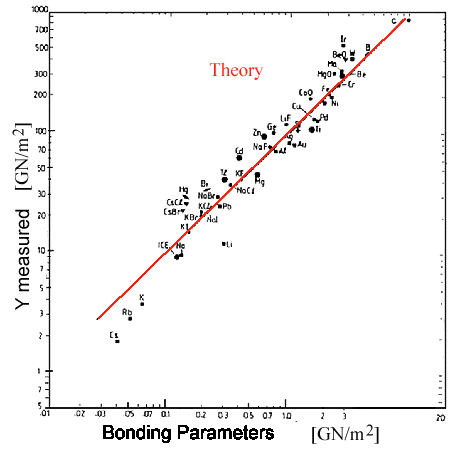Calculated Young's modulus (red line) and measured valuesThis is an old picture and I don't know who did it (sorry). It shows that the spring-bond theory is rather good and essentially demonstrates one thing:

 We have Young's modulus covered!Note that Young's modulus as determined from a tensile test measures the "springiness" of all bonds contained in a crystal. Since for low-alloy steels, the kind we consider for ancient swords, the overwhelming majority of the bonds are iron-iron bonds, Young's modulus of iron and all low -alloy steels must be pretty much the same, as claimed in the backbone text and found in experiments.

Melting Point and Thermal ExpansionIf you look at the first potential well picture again, you realize that all it takes to break the two atoms apart is to raise the energy level of the oscillations all the way up to the base line. The energy is now equal to the binding energy U0, and at the maximum distance between the atoms can become arbitrarily large. In this case you just as well can consider the atoms to be separated. They don't feel much attraction anymore, and if there are other atoms around that are perhaps closer, they go for other partners for a short time until they separate again.In other words, the material melts as soon as the vibration energy EVib approaches the binding energy and that gives us the melting point Tm. Since the vibration energy is nothing but a measure of the temperature T or the thermal energy kT (k = Boltzmann constant), we have kTm » U0That's a good general relation but not very precise when it comes to numbers. The reason is that we don't have just two but many atoms vibrating away. Moreover, melting doesn't start everywhere in a crystal (as it would in the consideration here) but always on the surface, where the conditions are different from the inside.
So I won't derive numbers from that consideration. Nevertheless, it gives a good general feeling for melting points.When materials get hot their dimension change because they expand. Not much, but enough to cause all kinds of massive engineering problems. The thermal expansion coefficient a characterizes this material property, it is simply the strain etherm caused by a temperature change of DT per Kelvin, i.e. a = etherm/DTWhy is there thermal expansion at all? Because the potential well is always asymmetric; it gets steeper at small distances and flatter for larger ones. The average distance of the vibrating atoms thus becomes a bit larger with increasing energy = temperature or amplitude; the material expands. This is already shown in the potential well figure above. All we need to do is to calculate the green line, defining the halfway points between the two sides of the potential well.
It is easy enough to do it geometrically so it should also be easy to do the algebra, starting from the general potential formula? No, that is actually rather tricky, The result, however, is simple

a  =   n + m + 3
»  const.  ·    1
2 · n · m · U0 TmThe essential point is that a is proportional to 1/U0 and thus approximately also to 1/Tm. The rest is some constant that is specific for a given material but very roughly about the same for most materials. Using that for comparing theory and experiment gives the figure below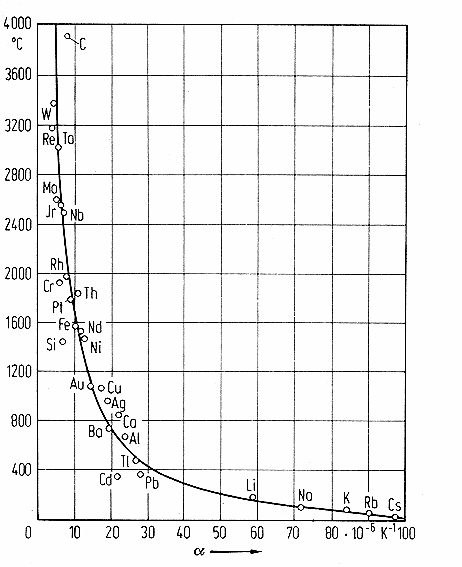Calculated and measured thermal expansion coefficientsOnce more a convincing result with the verdict:

 We have thermal expansion covered

Vibration Frequency of Atoms in a SolidThe potential well figures do not show the vibration frequency directly. However, even if you have no idea about physics, you know that if you jiggle some mass m on a spring with a spring constant ks, the vibration induced will be slower for large masses on the same spring and faster for the same mass if you take a "tougher" spring, i.e. a spring with a larger spring constant. Think of the wheels of your car on their suspension springs, for example.So we might guess that the vibration frequency n should be proportional to ks/m. Nice try - but not quite right. The precise relation is: n = 1/2p · (ks/m)½. So the proportionality is to the square root of ks/mSubstituting Young's modulus for ks we get

n  = æ ç è ö ÷ øPutting in some numbers for Young's modulus Y and interatomic distances r0 we get a very important rough number:

 The vibration frequency of atoms in a crystal is roughly 1013 HzLet's put that number into perspective:
• Operating frequency of your processor up there (called brain): around 50 Hz. Less after a few beers.
• Frequency of alternating line current: 50 Hz / 60 Hz.
• Frequency of radio waves: 100 kHz - some MHz
• Operating frequency of cell phones: around 1 GHz = 109 Hz.
• Operating frequency of a fast PC or Notebook: about 5 GHz.
• Operating frequency of your microwave oven: 8 Ghz
• Radar, other microwave stuff: up to an beyond 100 GHz.
• Body scanners at airports: a few THz = 1012 Hz
• Deep infrared radiations: (1012 - 1014 ) Hz
• Visible light: around 1015 Hz
• Ultraviolet light: 1016 HzThe little buggers move back and forth rather quickly, it seems. And that is the reason why atoms can cover distances far, far larger than their size when they move around by diffusion in a crystal. They cover only a tiny distance when they happen to jump into a vacancy - but they do that many times per second.

Ultimate Fracture StressThe last thing to consider is the ultimate fracture stress. Applying enough force or stress, or investing enough energy, will pull the atoms apart to any distance or simply apart. The material typically fractures if you increase the distance between atoms by 30 %As far as force is concerned, you only have to move the atoms apart to the point of inflection of the potential curve. At that point you need to apply the maximum force. If you want to pull the atoms apart even more, less force will be sufficient.
That is directly clear form looking at the force curve in this figure. The force is essentially the derivative of the potential curve. Where it has a maximum, its first derivative, and therefore also the second derivative of the potential curve, needs to be zero. That defines the point of inflection of the potential curve and if we calculate that we get the ultimate fracture stress.What we get after rather lengthy calculations is complicated - but not very useful:

 sfracture  =   n · m · n + 1 1/(n – m) U0 r03 æ ç è æ ç è ö ÷ ø ö ÷ ø

 efracture  · 100 »  30 %I will not compare that to experiments because this equation gives numbers far higher than what we measure. That's not because it is wrong but because it describes a perfect ideal and brittle crystal, something that doesn't exist. Moreover, it ruptures all the bonds in a cross-section together in one fell swoop, while in reality fracture occurs be a crack sweeping across the material and thus bonds are broken "one-by-one", something easier to do than to cut them all at the same time.
Moreover, the calculation here does not account for plastic deformation occurring with ductile crystals, that tends to reduce stresses. It simply does not "know" defects.
Fracture in real brittle crystals, however, is dominated by the defects I called "nanocracks". It therefore always happens at stresses far lower than the ultimate fracture stress calculated here for ideal perfect crystals; see this linkNevertheless, there is some merit in this consideration. It tells you what can be achieved under ideal conditions and thus it also tells you that there is no such thing as a "super" material that never breaks.Well, tough luck here. We do not have fracture covered by working with the bond-spring model. We can, however, still use it in a different kind of way to make some leeway in this respect. That is the topic of another module.

Famous Last WordsThe bond-spring model as expressed in the "master" equation above is an extremely simple and relatively old model. One could work it just with paper an pencil, and it produced important general results. It is not good enough, however, for serious Material Science for two major reasons:
1. The potential equation is far too simple. It does not, for example, account for the directionality of bonds.
2. Looking at just two atoms does not quite do justice to a crystal with a hell of a lot of atoms.Repairing those problems is simple but the resulting equations will not be amenable to tackling with paper and pencil. You need computers. You actually need rather powerful computers for tackling many of the more interesting questions. That's why computational materials science is making leaps and bounds in the last 10 years and is sure to make major contribution in years to come.In essence, however, we still first supply the potential describing the interaction between the atoms of the system and then go on from there. Here is a potential from a 2004 paper (couldn't resist), describing the interaction potentials between iron and phosphorous for all three possible variants: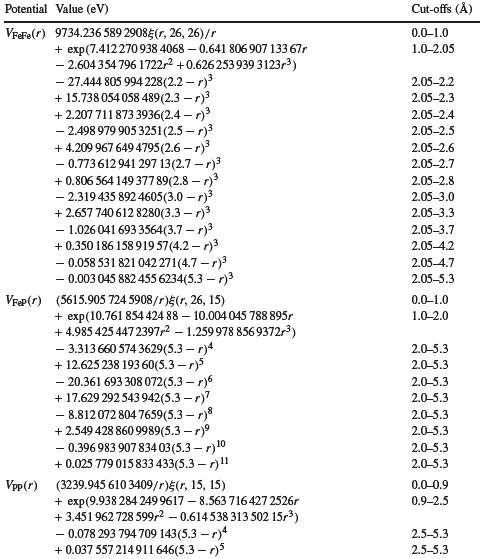Some "real" potentials used for calculations Source: G J Ackland, M IMendelev, D J Srolovitz, S Han and A V Barashev, Development of an interatomic potential for phosphorus impurities in a-iron, J. Phys.: Condens. Matter 16 (2004) S2629-S2642This somewhat lengthy module was meant to give a taste treat of what's going on in a small part of what we call the theory of materials. If you got the impression that we guys know what we are doing most of the time, I will rest my case.Periodic Table of the Elements3.1.2 Stiff or Hard?Fracture Mechanics IScience of Welding SteelBoltzmann DistributionAlloying Elements in DetailDislocation Science - 1. The BasicsScience of Uniaxial DeformationUnits and Constants4.4.2 Moving Atoms AroundExperimental Techniques for Measuring Diffusion ParametersWaves4.1.3 Young's Modulus and BondingCrystal Models6.1.3 Reading Phase Diagrams: Mixed Phases and BoundariesSteel PropertiesAtomic Mechanisms of DiffusionThermal StressThe Second Law12.2.5 Sword Types and Static Properties

© H. Föll (Iron, Steel and Swords script)